# Finding Single Missing Element in an Array in C

## Finding Single Missing Element in a Sorted Array in C:

In this article, we will see how to find out a missing element in a sequence of elements i.e. Finding Single Missing Element in a Sorted Array in C with Examples. Please read our previous article, where we have created a single Menu Driven Program that includes all the operations of an array.

##### Finding Single Missing Element in a Sorted Array

If the elements are stored in an array, then we have to find:

1. The single missing element in the sorted array.
2. Multiple missing elements in the sorted array.
3. Missing elements in an unsorted array.

There can be more than one possible way to find the missing element. So, let’s look at 1st method.

##### 1st Method: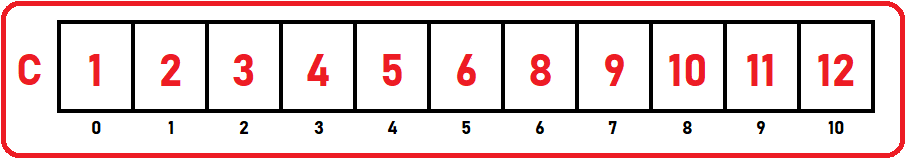Here we have taken an example of an array of size 11. These are first ‘n’ natural numbers. So, the sequence is starting from 1 onwards. If you noticed the above array, 7 is the missing element. Now we have to find out that 7 is missing in the above sequence.

We know the formula for the first n natural number which is: n (n+1) / 2. So, if I have a total of 12 elements that is the last element, we should know already about the last element. The sum of the first n natural number can be known by using – n (n+1) / 2. We can use this formula if we know the first and the last element. So, the sum is: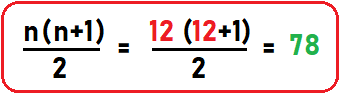So, the total of all the elements which are present in the array should be 78. If it is not 78, how much less it is, that will be our missing element. So, we have calculated the sum with the formula, now we add each element of the array and subtract that number from 78. Let’s see.

Below is the pseudo-code for finding the sum of each element in the array by the iterative method:

##### Pseudo Code:
```sum = 0;
for (int i = 0; i < 11; i++){
sum = sum + C [i]
}
```

Now we have the sum of the array in our sum variable. So now how to calculate the missing element? We have to subtract sum from above 78 (sum of 1st 12 natural number).

Missing Element = 78 – sum

= 78 – 71

= 7

So, this is the 1st method to find the single element in a sorted array.

##### Full Code in C language:
```#include <stdio.h>
#include <stdlib.h>

struct List{
int C;
int size;
int length;
};

void Display(struct List list) {
int i;
printf("Elements are:\n");
for (i = 0;i<list.length;i++)
printf("%d ", list.C[i]);
printf("\n\n");
}

// Valid only for single missing element for sum of first n natural numbers
void MissingElements(struct List list, int max){

int calcSum = max * (max + 1) / 2;

int listSum = 0;

for(int i = 0; i < list.length; i++){
listSum += list.C[i];
}

printf("Missing Elements is: %d", calcSum - listSum);
}

int main(){
struct List list_1 = {{1, 2, 3, 4, 5, 6, 8, 9, 10, 11, 12}, 15, 11};

Display(list_1);

MissingElements(list_1, 12);
}
```
###### Output: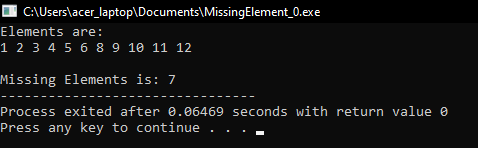##### 2nd Method:

Here the missing element is not in the first n natural number. The sequence may be starting from any point.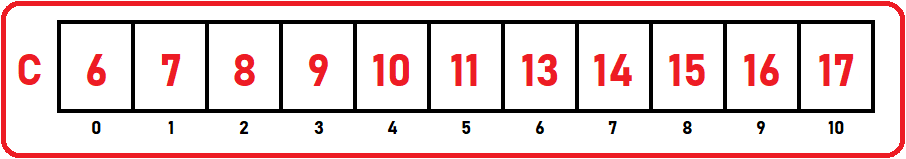Here the starting number of arrays is 6 and the last number is 17 and these are a total of 11 numbers (0 to 10). This is not the sum of 1st n natural number. First, we have to know the first and last elements which are 6 and 17. In this array, 12 is the missing element.

So, we have the lowest number, highest number, and the number of elements as L = 6, H = 17, N = 11. Here we will find the missing elements with the help of indices. Difference between:

1st element and 0th index is: 6 – 0 = 6.

2nd element and 1st index is: 7 – 1 = 6.

3rd element and 2nd index is: 8 – 2 = 6.

4th element and 3rd index is: 9 – 3 = 6.

5th element and 4th index is: 10 – 4 = 6.

6th element and 5th index is: 11 – 5 = 6.

7th element and 6th index is: 13 – 6 = 7.

Here the common difference is 6 and at the 6th index, we got a difference of 7. So here is the missing element. So, what should be the missing element? We know the difference is 6. So we have to add a common difference with the index number where the element is missing:

Difference + index number = missing element.

6 + 6 = 12

Now we got the missing element which is 12. So, the logic is simple, we have to stop at that index where we found the change in the difference of element and indices.

##### Pseudo Code:
```difference = low – 0;
for (i = 0; i < n; i++){
if (C [i] – i != difference){
printf (“Missing element %d\n”, i + difference);
break;
}
}
```

Time Complexity: O (n)

##### Full Code in C language:
```#include <stdio.h>
#include <stdlib.h>

struct List{
int C;
int size;
int length;
};

void Display(struct List list) {
int i;
printf("Elements are:\n");
for (i = 0;i<list.length;i++)
printf("%d ", list.C[i]);
printf("\n\n");
}

void MissingElements(struct List list){

int difference = list.C - 0;

for (int i = 0; i < list.length; i++){
if (list.C [i] - i != difference){
printf ("Missing element is: %d\n", i + difference);
break;
}
}
}

int main(){
struct List list_1 = {{6, 7, 8, 9, 10, 11, 13, 14, 15, 16, 17}, 15, 11};

Display(list_1);

MissingElements(list_1);
}
```
###### Output: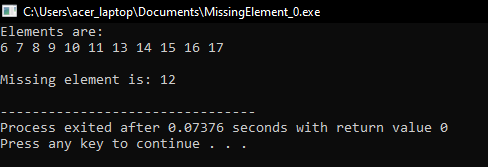Here we already know the missing element is 12 only so we stop at the index where we found the missing element. But if we want to find multiple elements we will continue to the end of the array. We will see that in our next article.

In the next article, I am going to discuss Finding Multiple Missing Elements in a Sorted Array in C Language with Examples. Here, in this article, I try to explain How to Find a Single Missing Element in a Sorted Array in C with Examples and I hope you enjoy this Finding Single Missing Element in a Sorted Array in C with Examples article.